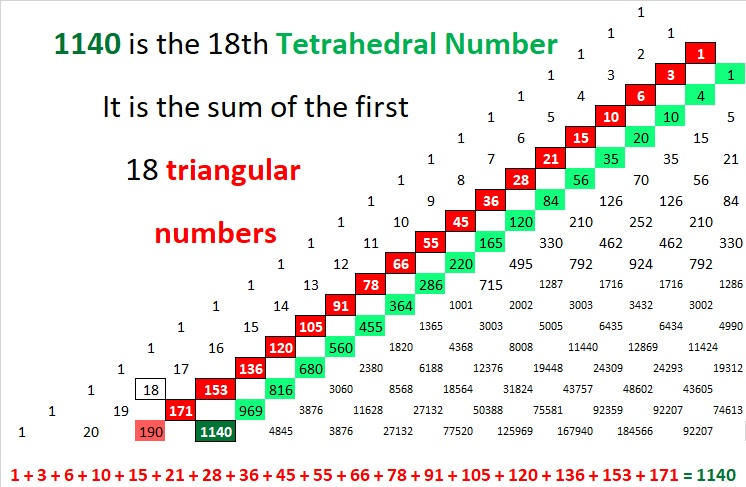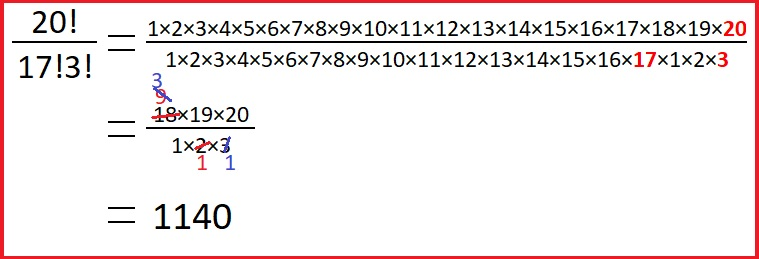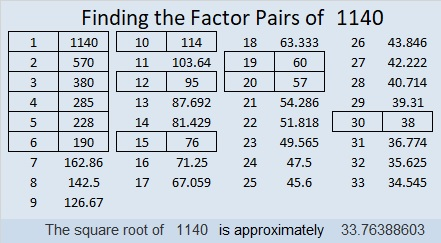# 1140 is the 18th Tetrahedral Number

1140 is the 18th tetrahedral number because it satisfies this formula:
(18)(18+1)(18+2)/6 = 1140

It is the 18th tetrahedral number because it is the sum of the first 18 triangular numbers:Since 18 is an even number, 1140 is the sum of the first 9 EVEN squares.

If the 1140 tiny squares in that graphic were cubes, they could be stacked into a tower with either a triangular base or a square base. Then we would see the beauty of this tetrahedral number.

We can see the number 1140 as well as ALL the previous tetrahedral numbers on this portion of Pascal’s Triangle. (They are the green squares.):1140 has its place as the 3rd number (as well as the 17th number) on the 20th row of Pascal’s triangle because of this next formula:Here are some other facts about the number 1140:

• 1140 is a composite number.
• Prime factorization: 1140 = 2 × 2 × 3 × 5 × 19, which can be written 1140 = 2² × 3 × 5 × 19
• The exponents in the prime factorization are 2, 1, 1, and 1. Adding one to each and multiplying we get (2 + 1)(1 + 1)(1 + 1)(1 + 1) = 2 × 3 × 2 × 2 = 24. Therefore 1140 has exactly 24 factors.
• Factors of 1140: 1, 2, 3, 4, 5, 6, 10, 12, 15, 19, 20, 30, 38, 57, 60, 76, 95, 114, 190, 228, 285, 380, 570, 1140
• Factor pairs: 1140 = 1 × 1140, 2 × 570, 3 × 380, 4 × 285, 5 × 228, 6 × 190, 10 × 114, 12 × 95, 15 × 76, 19 × 60, 20 × 57, or 30 × 38,
• Taking the factor pair with the largest square number factor, we get √1140 = (√4)(√285) = 2√285 ≈ 33.76389Here are some factor trees that use 11 of 1140’s factor pairs:1140 is the sum of consecutive prime numbers two different ways:
179 + 181 + 191 + 193 + 197 + 199 = 1140,
569 + 571 = 1140

1140 is the hypotenuse of a Pythagorean triple:
684-912-1140 which is (3-4-5) times 228

1140 looks interesting when it is written in a couple other bases:
It’s palindrome 474 in BASE 16 because 4(16²) + 7(16) + 4(1) = 1140,
and it’s 330 in BASE 19 because 3(19²) + 3(19) = 3(19² + 19) = 3(19)(20) = 1140

This site uses Akismet to reduce spam. Learn how your comment data is processed.